### 二、一般情形的线性方程组

n个未知量m个方程的线性方程组取如下形式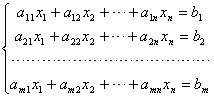1

A=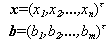Ax=b 1

Ax=0 2

A称为方程组（1）的系数矩阵，

C=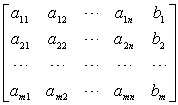[线性方程组有解的判别定理] r(A),r(C)分别表示系数矩阵A与增广矩阵C的秩，则有

1° 当m=nr(A)= r(C)=n（或ôAô ¹ 0）时，方程组（1）有唯一解；

2° 当r(A)< r(C)时，方程组（1）无解，这时（1）称为矛盾方程组；

3° 当r(A)= r(C)=r<n（或ôAô =0）时，方程组（1）有无穷多组解；

4° 齐次线性方程组（2）有非零解的充分必要条件是：r(A)<n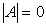.

[线性方程组的解的结构]

1°r(A)=r<n时，齐次方程组Ax=0的任一非零解x=(x1,x2,...,xn)t都可用它的n?r个线性无关解x(i)=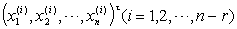的线性组合来表示.

n-r个线性无关解称为方程组的基础解系，它不是唯一的.

2x(0)=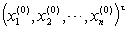是线性方程组Ax=b的一个特解，则它的任一解x=(x1,x2,...,xn)t 都可以表示为

x=x(0)+h